# Maharashtra Board Class 10 Science Solutions Part 1 Chapter 4 Effects of Electric Current

Balbharti Maharashtra State Board Class 10 Science Solutions Part 1 Chapter 4 Effects of Electric Current Notes, Textbook Exercise Important Questions and Answers.

## Maharashtra State Board Class 10 Science Solutions Part 1 Chapter 4 Effects of Electric Current

Class 10 Science 1 Chapter 4 Effects Of Electric Current Exercise Question 1.
Tell the odd one out. Give proper explanation.
a. Fuse wire, bud conductor, rubber gloves, generator.
Generator. It converts mechanical energy into electric energy, the remaining three do not.

b. Voltmeter, Ammeter, gulvanometer, thermometer.
Thermometer. It measures temperature, the remaining three measure electrical quantities.

c. Loud speaker, microphone, electric motor, magnet.
Magnet. It exerts a force on a magnetic material, the remaining three convert one form of energy into another.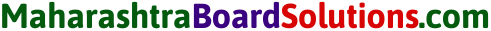4 Effects Of Electric Current Exercise Question 2.
Explain the construction and working of the following. Draw a neat diagram and label it.
a. Electric motor
Figure shows the construction of an electric motor. Here, a rectangular loop ABCD of copper wire with resistive coating is placed between the north pole and south pole or a strong magnet, such as a horseshoe magnet, such that the branches AB and CD are perpendicular to the direction of the magnetic field. The ends of the loop are connected to the two halves, X and Y, of split rings X and Y have resistive coating on their inner surfaces and are tightly fitted on the axle. The outer conducting surfaces of X and Y are in contact with two stationary carbon brushes, E and F, respectively.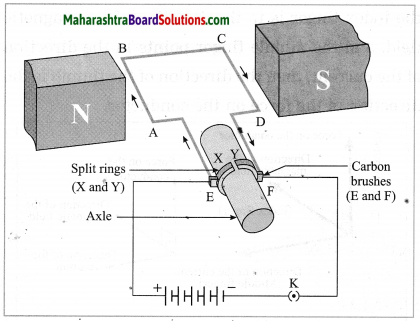Working:
1. When the circuit is completed with a plug key or switch, the current flows in the direction E → A → B → C → D → F. As the magnetic field is directed from the north pole to the south pole, the force on AB is downward and that on CD is upward by Fleming’s left hand rule. Hence, AB moves downward and CD upward. These forces are equal in magnitude and opposite in direction. Therefore, as observed from the side AD, the loop ABCD and the axle start rotating in anticlockwise direction.

2. After half a rotation, X and Y come in contact with brushes F and E respectively and the current flows in the direction EDCBAF. Hence the force on CD is downward and that on AB is upward. Therefore, the loop and the axle continue to rotate in the anticlockwise direction.

3. After every half rotation, the current in the loop is reversed and the loop and the axle continue to rotate in anti clockwise direction. When the current is switched off, the loop stops rotating after some time.

b. Electric Generator (AC)
Figure shows the construction of an AC electric generator. Here, a coil ABCD of copper wire is kept between the pole pieces (N and S) of a strong magnet. The ends of the coil are connected to the conducting rings R1 and R2 via carbon brushes B1 and B2. The rings are fixed to the axle and there is a resistive coating in between the rings and the axle. The stationary brushes are connected to a galvanometer used to show the direction of the current in the circuit.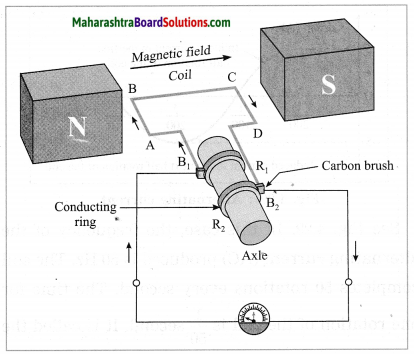Working:
When the axle is rotated with a machine from outside, the coil ABCD starts rotating. Suppose the coil rotates in clockwise direction, as observed from the side AD. Then as the branch AB moves upward, the branch CD moves downward. By Fleming’s right hand rule, the induced current flows in the direction A → B → C → D and in the external circuit, it flows from B2 to B, through the galvanometer. The induced current is proportional to the number of turns of the copper wire in the coil.

After half a rotation, AB and CD interchange their places. Hence, the induced current flows in the direction D → C → B → A. As AB is always in contact with B1 and CD is in contact with B2, the current in the external circuit flows from B1 to B2 through the galvanometer. Thus, the direction of the current is the external circuit is opposite to that in the previous half rotation. The process goes on repeating and alternating current is generated.4 Effects Of Electric Current Question 3.
Electromagnetic induction means
a. Charging of an electric conductor.
b. Production of magnetic field due to a current flowing through a coil.
c. Generation of a current in a coil due to relative motion between the coil and the magnet.
d. Motion of the coil around the axle in an electric motor.
c. Generation of a current in a coil due to relative motion between the coil and the magnet.

Electric Current Question 4.
4. Explain the difference: AC generator and DC generator.
AC generator:

1. In an AC generator, the rings used are not split.
2. The direction of the current produced reverses after equal intervals of time.

DC generator:

1. In a DC generator, split rings are used.
2. The current produced flows in the same direction all the time.

Question 5.
Which device is used to produce electricity? Describe with a neat diagram.
(1) Electric motor
(2) Galvanometer
(3) Electric generator (DC)
(4) Voltmeter
Electric generator (DC).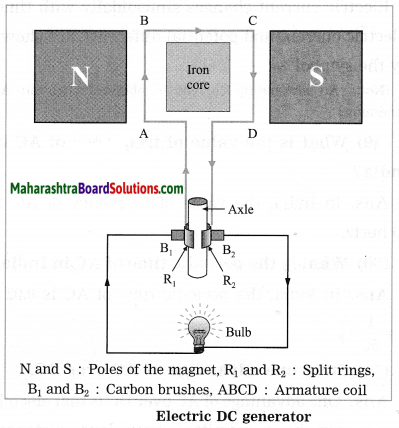Figure shows the construction of a DC generator.
Working: The axle is rotated with a machine from outside. When the armature coil of the generator rotates in the magnetic field, electric potential difference is produced in the coil due to electromagnetic induction. This produces a current as shown by the glowing of the bulb or by a galvanometer. The direction of the current depends on the sense of rotation of the coil.

In a DC generator, one brush is always in contact with the arm of the coil moving up while the other brush is in contact with the arm of the coil moving down in the magnetic field. Hence, the flow of the current in the circuit is always in the same direction and the current flows so long as the coil continues to rotate in the magnetic field.

[Note In the case of a DC generator, the current is in the same direction during both the halves of the rotation of the coil. The magnitude of the current does vary periodically with time. In this respect, it differs from the current supplied by an electric cell.]

Question 6.
How does the short circuit form? What is its effect?
If a bare live wire (phase wire) and a bare neutral wire touch each other (come in direct contact) or come very close to each other, the resistance of the circuit becomes very small and hence huge (very high) electric current flows through it. This condition is called a short circuit or short circuiting.

In this case, a large amount of heat is produced and the temperature of the components involved becomes very high. Hence, the circuit catches fire.Question 7.
Give scientific reasons:
a. Tungsten is used to make a solenoid type coil in an electric bulb.
1. The intensity of light emitted by the filament of a bulb depends on the temperature of the filament. It increases with the temperature.

2. The melting point of the material used to make the filament of a bulb should be very high so that the filament can be heated to a high temperature by passing a current through it, without melting it. This enables us to obtain more light. The melting point of tungsten is very high.

Hence, tungsten is used to make a solenoid type coil (filament) in an electric bulb.

b. In the electric equipment producing heat e.g. iron, electric heater, boiler, toaster, etc. an alloy such as Nichrome is used, not pure metals.
1. The working of heating devices such as a toaster and an electric iron is based on the heating effect of electric current, i.e., conversion of electric energy into heat by passage of electric current through a metallic conductor.

2. An alloy, such as Nichrome, has high resistivity and it can be heated to a high temperature without oxidation, in contrast to pure metals. Therefore, the coils in heating devices such as a toaster and an electric iron are made of an alloy, such as Nichrome, rather than a pure metal.

c. For electric power transmission, copper or aluminium wire is used.
1. Copper and aluminium are good conductors of electricity.

2. Copper, and aluminium have very low resistivity. Hence, when an electric current flows through a wire of copper or aluminium, heat produced is comparatively low. Therefore, for electric power transmission, copper or aluminium wire is used.

d. In practice the unit kWh is used for the measurement of electric energy, rather than the joule.
(1) If an electric device rated 230 V, 5 A is operated for one hour, electric energy used
= VIt = 230 V × 5 A × 3600 s = 4140000 joules.

(2) If this energy is expressed in kW.h, it will be $$\frac{4140000}{3.6 \times 10^{6}}$$ kW·h = 1.15 kW·h (more convenient). 3.6 × 106
Hence, in practice the unit kW·h is used for the measurement of electric energy, rather than the joule.

Question 8.
Which of the statements given below correctly describes the magnetic field near a long, straight current-carrying conductor?
(1) The magnetic lines of force are in a plane, perpendicular to the conductor in the form of straight lines.
(2) The magnetic lines of force are parallel to the conductor on all the sides of conductor.
(3) The magnetic lines of force are perpendicular to the conductor going radially outward.
(4) The magnetic lines of force are in concentric circles with the wire as the center, in a plane perpendicular to the conductor.
The magnetic lines of force are in concentric circles with the wire as the centre, in a plane perpendicular to the conductor.Question 9.
What is a solenoid? Compare the magnetic field produced by a solenoid with the magnetic field of a bar magnet. Draw neat figures and name various components.
When a copper wire with a resistive coating is wound in a chain of loops (like a spring), it is called a solenoid.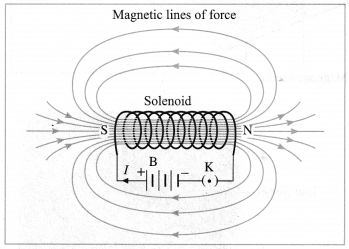Magnetic lines of force (magnetic field lines) due to a current carrying solenoid.
B: Battery, K: Plug key, I: Current, N: North pole, S: South pole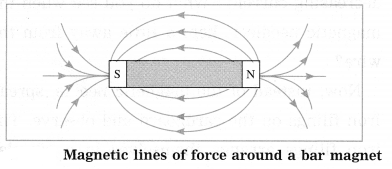The magnetic field lines (magnetic lines of force) due to a current-carrying solenoid are similar to those of a bar magnet. One face of the coil acts as the south pole and the other face as the north pole.

[Note: A current-carrying coil, like a magnet, can be used to magnetise the rod of a given material such as carbon steel or chromium steel. With a strong megnetic field, permanent magnetism can be produced in these materials.]

Question 10.
Name the following diagrams and explain the concept behind them.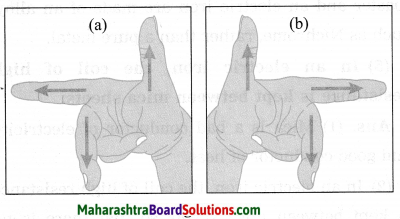(a) Fleming’s right hand rule:
Stretch the thumb, the index finger and the middle finger of the right hand in such a way that they are perpendicular to each other. In this position, the thumb indicates the direction of the motion of the conductor, the index finger the direction of the magnetic field, and the middle finger shows the direction of the induced current.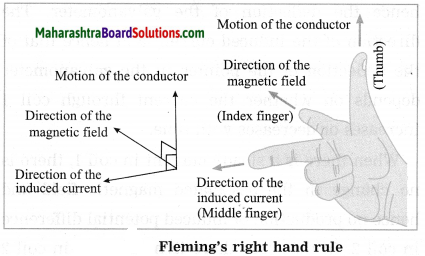[Note The induced current is maximum when the direction of motion of the conductor is at right angles to the magnetic field. ]

(b) Fleming’s left hand rule: The left hand thumb, index finger, and the middle finger are stretched so as to be perpendicular to each other. If the index finger is in the direction of the magnetic field, and the middle finger points in the direction of the current, then the direction of the thumb in the direction of the force on the conductor.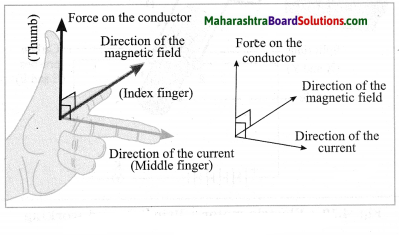[Note: A magnetic field exerts a force on a current-carrying conductor. Electric current is the time rate of flow of electric charge. Thus, a magnetic field exerts a force on a moving charge. This property is used to accelerate charged particles such as protons, deuterons and alpha particles, as well as electrons, to very high energies. A machine used for this purpose is called a charged particle accelerator. It may be linear or circular in design and very big in size. Such high energy particles are used to study the structure of matter. ]Question 11.
Identify the figures and explain their use.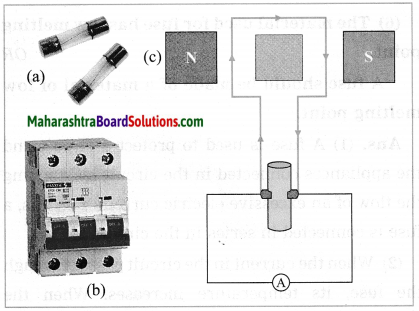(a) Fuse:
A fuse protects electrical circuits and appliances by stopping the flow of electric current when it exceeds a specified value. For this, it is connected in series with the appliance (or circuit) to be protected. A fuse is a piece of wire made of an alloy of low melting point (e.g. an alloy of lead and tin). If a current larger than the specified value flows through the fuse, its temperature increases enough to melt it. Hence, the circuit breaks and the appliance is protected from damage.

[Note : The fuse wire is usually enclosed in a cartridge of an insulator such as glass or porcelain provided with metal caps. The current rating (such as 1 A, 2 A) may be printed on the cartridge. ]

(b) Miniature circuit breaker:
These days miniature circuit breaker (MCB) switches are used in homes. When the current in the circuit suddenly increases this switch opens and current stops. Different types of MCBs are in use. For the entire house, however the usual fuse wire is used.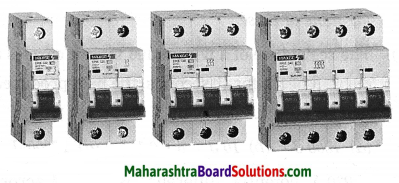(c) Figure shows the construction of a DC generator.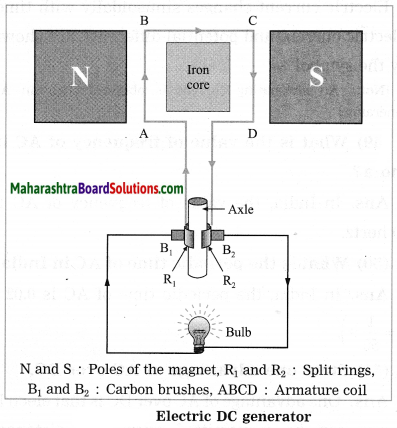Here, an ammeter is shown instead of a bulb.
Working: The axle is rotated with a machine from outside. When the armature coil of the generator rotates in the magnetic field, electric potential difference is produced in the coil due to electromagnetic induction. This produces a current as shown by the glowing of the bulb or by a galvanometer. The direction of the current depends on the sense of rotation of the coil.

In a DC generator, one brush is always in contact with the arm of the coil moving up while the other brush is in contact with the arm of the coil moving down in the magnetic field. Hence, the flow of the current in the circuit is always in the same direction and the current flows so long as the coil continues to rotate in the magnetic field.Question 12.
Solve the following examples.
a. Heat energy is being produced in a resistance in a circuit at the rate of 100 W. The current of 3 A is flowing in the circuit. What must be the value of the resistance?
Solution:
Data: P = 100 W, I = 3 A, R = ?, P = I2R
∴ Resistance, R = $$\frac{P}{I^{2}}=\frac{100 \mathrm{W}}{(3 \mathrm{A})^{2}}=\frac{100}{9} \Omega$$ = 11.11 Ω

b. Two tungsten bulbs of wattage 100 W and 60 W power work on 220 V potential difference. If they are connected in parallel, how much current will flow in the main conductor?
Solution:
Data : P1 = 100 W, P2 = 60 W, V = 220 V,
I = ?, ∴ I = $$\frac{P}{V}$$
P = VI
∴ I1 = $$\frac{P_{1}}{V}$$ and I2 = $$\frac{P_{2}}{V}$$
Current in the main conductor, I = I1 + I2 (parallel connection)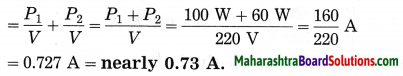c. Who will spend more electrical energy? 500 W TV set in 30 mins, or 600 W heater in 20 mins?
Solution:
Data : P1 = 500 W, t1 = 30 min = $$\frac{30}{60}$$ h
= $$\frac{1}{2}$$ h, P2 = 600 W, t2 = 20 min = $$\frac{20}{60}$$ h = $$\frac{1}{3}$$ h
Electrical energy used = Pt
TV set : P1t1 = 500 W × $$\frac{1}{2}$$ h = 250 W·h
Heater : P2t2 = 600 W × $$\frac{1}{3}$$ h = 200 W·h
Thus, the TV set will spend more electrical energy than the heater.

d. An electric iron of 1100 W is operated for 2 hours daily. What will be the electrical consumption expenses for that in the month of April? (The electric company charges ₹ 5 per unit of energy.)
Solution:
Data: P = 1100 W, t = 2 × 30 = 60 h,
₹ 5 per unit of energy, expenses = ?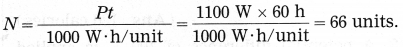∴ Electrical consumption expenses = 66 units × ₹ 5 per unit = ₹ 330.

Project:
Project 1.
Under the guidance of your teachers, make a ‘free-energy generator’.

Can you recall? (Text Book Page No. 47)

Question 1.
How do we decide that a given material is a good conductor of electricity or is an insulator?
A material which has very low electrical resistance is called a good conductor of electricity. Examples: silver, copper, aluminium.
A material which has extremely high electrical resistance is called an insulator of electricity. Examples: rubber, wood, glass.Question 2.
Iron is a conductor of electricity, but when we pick up a piece of iron resting on the ground, why don’t we get electric shock?
When we pick up a piece of iron resting on the ground, we don’t get electric shock because that piece does not carry any electric current at that time.

Use your brain power! (Text Book Page No. 48)

Question 1.
If in the circuit, the resistor is replaced by a motor, in which form will the energy given by the cell get transformed into?
The energy given by the cell will get transformed into the kinetic energy of the copper coil in the motor.

Use your brain power! (Text Book Page No. 60)

Question 1.
Draw the diagram of a DC generator. Then explain as to how the DC current is obtained.
Figure shows the construction of a DC generator.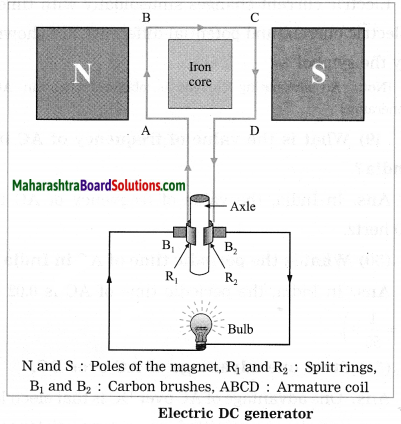Working:
The axle is rotated with a machine from outside. When the armature coil of the generator rotates in the magnetic field, electric potential difference is produced in the coil due to electromagnetic induction. This produces a current as shown by the glowing of the bulb or by a galvanometer. The direction of the current depends on the sense of rotation of the coil.

In a DC generator, one brush is always in contact with the arm of the coil moving up while the other brush is in contact with the arm of the coil moving down in the magnetic field. Hence, the flow of the current in the circuit is always in the same direction and the current flows so long as the coil continues to rotate in the magnetic field.

[Note In the case of a DC generator, the current is in the same direction during both the halves of the rotation of the coil. The magnitude of the current does vary periodically with time. In this respect, it differs from the current supplied by an electric cell.]

Fill in the blanks and rewrite the completed statements:

Question 1.
Electric power = V2/…….
Electric power = $$\frac{V^{2}}{R}$$

Question 2.
……….= 1 joule/1 second.
1 watt = 1 joule /1 second.

Question 3.
1 kW.h =………J.
1 kW.h = 3.6 x 106 J.

Question 4.
According to Joule’s law, quantity of heat (H) produced by an electric current =……….
According to Joule’s law, quantity of heat (H) produced by an electric current = I2Rt or VIt or $$\frac{V^{2}}{R}$$t

Question 5.
Magnetic effect of electric current was dicovered by………..
Magnetic effect of electric current was dicovered by Hans Christian Oersted.Question 6.
………..is expressed in oersted.
Intensity of magnetic field is expressed in oersted.

Question 7.
Electromagnetic induction was discovered by………..
Electromagnetic induction was discovered by Michael Faraday and independently by Joseph Henry.

Question 8.
A galvanometer is used for………
A galvanometer is used for detecting the presence of current in a circuit, as well as for some electrical measurements.

Question 9.
In India, the frequency of alternating current is……….
In India, the frequency of alternating current is 50 Hz or 50 cycles per second.

Question 10.
Electric motor converts electric energy into………energy.
Electric motor converts electric energy into mechanical energy.

Question 11.
Electric generator converts………..energy into electric energy.
Electric generator converts mechanical energy into electric energy.

Rewrite the following statements by selecting the correct options:

Question 1.
The device used for producing a current is called……….
(a) a voltmeter
(b) an ammeter
(c) a galvanometer
(d) a generator
(d) a generator

Question 2.
At the time of short circuit, the current in the circuit………
(a) increases
(b) decreases
(c) remains the same
(d) increases in steps
(a) increases

Question 3.
The direction of the magnetic field around a straight conductor carrying current is given by……
(a) the right hand thumb rule
(b) Fleming’s left hand rule
(c) Fleming’s right hand rule
(d) none of these
(a) the right hand thumb ruleQuestion 4.
The resistance of a wire is 100 Ω. If it carries a current of 1A for 10 seconds, the heat produced will be……….
(a) 1000 J
(b) 10 J
(c) 0.1 J
(d) 10000 J
(a) 1000 J

Question 5.
If 220 V potential difference is applied across an electric bulb, a current of 0.45 A flows in the bulb. What must be the power of the bulb? (Practice Activity Sheet – 1)
(a) 99 W
(b) 70 W
(c) 45 W
(d) 22 W
(a) 99 W

Question 6.
Electromagnetic induction means
(a) charging of an electric conductor.
(b) production of magnetic field due to a current flowing through a coil.
(c) generation of a current in a coil due to relative motion between the coil and the magnet.
(d) motion of the coil around the axle in an electric motor.
(c) generation of a current in a coil due to relative motion between the coil and the magnet.

Question 7.
Write the correct option by observing the figures. (Practice Activity Sheet – 2)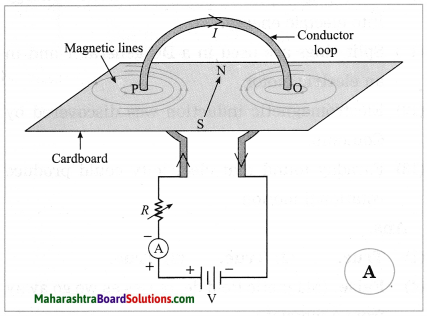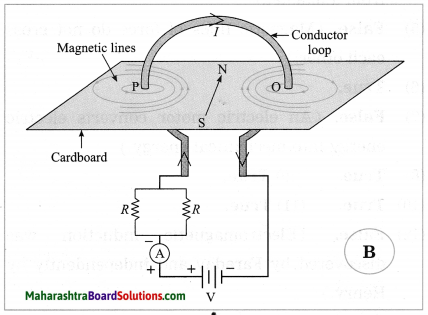(a) Magnetic field in A is stronger.
(b) Magnetic field in B is stronger.
(c) Magnetic fields in A and B are same.
(d) Magnetic fields in A and B are weaker.
(b) Magnetic field in B is stronger.
[Explanation : The resistance in circuit B is less (parallel combination) than that in A. Hence, the current in B is more than that in A. Therefore, the magnetic field in B is stronger than that in A.]

Question 8.
Observe the following diagram and choose the correct alternative: (March 2019)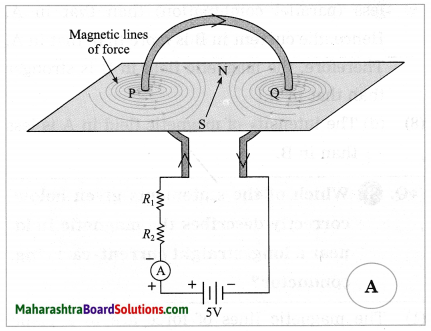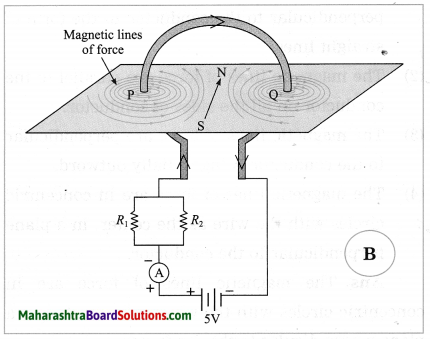(a) The intensity of magnetic field in A is larger than in B.
(b) The intensity of magnetic field in B is less than in A.
(c) The intensity of magnetic field in A and B is same.
(d) The intensity of magnetic field in A is less than in B.
(d) The intensity of magnetic field in A is less than in B.

State whether the following statements are true or false. (If a statement is false, correct it and rewrite it.) :

Question 1.
Electric power = I2R.
True.

Question 2.
Magnetic poles exist in pairs.
True.

Question 3.
Electromagnetism was discovered by Oersted.
True.Question 4.
Magnetic field increases as we go away from a magnet.
False. (Magnetic field decreases as we go away from a magnet.)

Question 5.
Magnetic lines of force cross each other.
False. (Magnetic lines of force do not cross each other.)

Question 6.
Electric generator is used to generate current.
True.

Question 7.
An electric motor converts mechanical energy into electric energy.
False. (An electric motor converts electric energy into mechanical energy.)

Question 8.
In India, the frequency of AC is 50 Hz.
True.

Question 9.
The electricity meter in the domestic electric circuit measures electrical energy consumption in kilowatt·hours.
True.

Question 10.
Electric generator converts mechanical energy into electric energy.
True.

Question 11.
Split rings are used in a DC generator and in an electric motor.
True.

Question 12.
Electromagnetic induction was discovered by Coulomb.
False. (Electromagnetic induction was discovered by Faraday and independently by Henry.)

Question 13.
Faraday found that electricity could produce rotational motion.
True.

Tell the odd one out. Give proper explanation:

Quesrtion 1.
Find the odd one out and justify it.
Fuse wire, M.C.B., rubber gloves, generator. (Practice Activity Sheet – 3)
Generator. It converts mechanical energy into electric energy. All others are related to safety measures to avoid mishap due to electricity.

Match the columns:

 Column I Column II 1. The right hand thumb rule a. The direction of the force on a current-carrying conductor placed in a magnetic field. 2. Fleming’s right hand rule b. The direction of the magnetic field around a straight conductor carrying a current. 3. Fleming’s left hand rule c. The direction of induced current in a conductor.

(1) The right hand thumb rule – The direction of the magnetic field around a straight conductor carrying a current.
(2) Fleming’s right hand rule – The direction of induced current in a conductor.
(3) Fleming’s left hand rule – The direction of the force on a current-carrying conductor placed in a magnetic field.Name the following:

Question 1.
The negatively charged particle considered as a free particle moving in a metallic conductor.
Electron.

Question 2.
The quantity expressed in ampere.
Electric current.

Question 3.
The quantity expressed in ohm.
Electric resistance.

Question 4.
The quantity expressed in volt.
Electric potential.

Question 5.
The quantity expressed in joule.
Work (and energy).

Question 6.
The quantity expressed in watt.
Power.

Question 7.
The quantity expressed in kilowatt-hour.
Electric energy.

Question 8.
A component used to control the current.
Resistor.

Question 9.
An instrument used to measure electric current.
Ammeter

Question 10.
An instrument used to measure electric potential difference.
Voltmeter.

Question 11.
The ratio of the work done to the quantity of charge transferred.
Electric potential difference.

Question 12.
An alloy of Ni, Cr, Mn and Fe.
Nichrome.

Question 13.
The SI unit of resistance.
The ohm.

Question 14.
A metal used to make the filament of an electric bulb.
Tungsten.

Question 15.
An alloy used to prepare a coil of high resistance for use in electric appliances such as an electric heater.
Nichrome.

Question 16.
Constituents of the alloy used to make a fuse wire.

Question 17.
The unit same as the watt·second.
The joule.

Question 18.
A unit for intensity of magnetic field.
The oersted.

Question 19.
The scientist in whose honour the SI unit of power is named.
James WattQuestion 20.
A device that converts electric energy into mechanical energy.
Electric motor.

Question 21.
A device that converts mechanical energy into electric energy.
Electric generator.

Answer the following questions in one sentence each :

Question 1.
What is the production of magnetism by an electric current called?
The production of magnetism by an electric current is called electromagnetism.

Question 2.
Is magnetic field a scalar or a vector?
Magnetic field is a vector.

Question 3.
In India, what is the time interval in which AC changes direction?
In India, AC changes direction every $$\frac{1}{100}$$ s.

Question 4.
What is the periodic time of AC in India?
In India, the periodic time of AC $$\frac{1}{50}$$ is

Question 1.
Define electric power.
Electric power is the electric work done per unit time.
OR
Electric power is the rate at which electric energy is used.

Question 2.
State the formula for electric power. Hence, obtain its SI unit.
Electric power (P) =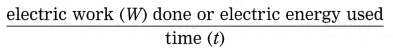The SI unit of work is the joule and that of time is the second. Hence, the SI unit of power is the joule per second. It is given the special name: the watt (W). One watt equals one joule per second.
W = VIt = I2Rt = $$\frac{V^{2}}{R}$$ t
∴ P = W/t = VI = I2R = V2/R.
Here, V is the potential difference applied across an electrical appliance, R is the resistance of the appliance and I is the current through the appliance.

[ Note The SI unit of power, the watt, is named in honour of James Watt (1736-1819), British instrument maker and engineer. ]Question 3.
What is the commercial unit of electric energy? Obtain the relation between this unit and the SI unit of energy.
The commercial unit of electric energy is the kilowatt·hour (kW·h) and the SI unit of energy is the joule (J).
1 kW·h = 103 $$\frac{J}{s}$$ × 3600 s s
= 3.6 × 106 J
[Note: The kilowatt-hour is often called simply the unit. (See the energy bill, i.e., the electricity bill.)]

Question 4.
What is one kilowatt.hour?
One kilowatt-hour is the electric energy used in one hour by an electrical appliance of power one kilowatt. It is equal to 3.6 × 106 J.

Question 5.
What is heating effect of electric current? What is its origin?
The production of heat in a resistance due to the electric current flowing through it when it is connected in an electrical circuit, is called the heating effect of electric current.

When a potential difference is applied across a metallic conductor, free electrons in the conductor move from the end at the lower potential to the end at the higher potential giving rise to electric current. These electrons collide with the atoms and positive ions and transfer some kinetic energy to them. This energy is converted into heat. Hence, the temperature of the conductor begins to rise i.e., the conductor becomes hot. This is the origin of the heating effect of electric current.

Question 6.
Statement 1: Electric current (flow of electrons) creates heat in a resistor.
Statement 2: Heat in the resistor is created according to the law of energy conservation.
Explain Statement 1 with the help of Statement 2. (Practice Activity Sheet – 2)
(1) When electrons flow through a resistor (during flow of electric current) electrons possess kinetic energy.
(2) During the flow of electrons there is a decrease in the kinetic energy of the electrons due to collisions with atoms, ions and molecules.
(3) According to the law of conservation of energy, this decrease in the kinetic energy of the electrons gets converted into heat.

Question 7.
State Joule’s law about heating effect of electric current.
Joule’s law about heating effect of electric current: The quantity of heat produced in a conductor when a current flows through it is directly proportional to (1) the square of the current (2) the resistance of the conductor (3) the time for which the current flows.Question 8.
Obtain the mathematical expression for the heat generated in a metallic conductor by electric current (Joule’s law).
If V is the potential difference applied across a metallic conductor of resistance R, the current through the conductor, given by Ohm’s law, is
I = V/R ……(1)
The charge passing through the conductor in time t when the current I flows in the conductor is
Q = It…….(2)
The work done in this process is W = VQ …..(3)
From Eqs. (1), (2) and (3), we have,
W = (IR) (It) = I2Rt = VIt
= $$V\left(\frac{V}{R}\right) t=\frac{V^{2}}{R} t$$
This work is converted into heat.
When I is expressed in ampere, R in ohm, t in second and V in volt, W is expressed in joule. In that case,
W = I2Rt = VIt = $$\frac{V^{2}}{R}$$ t (in joule)
Usually heat energy (H) is expressed in calorie. Using the relation 4.18 J = 1 cal, we have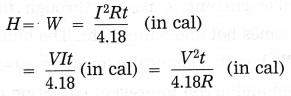This is the required equation.

Question 9.
Two dissimilar bulbs are connected in series. Which bulb will be brighter? (Hint: Consider the resistance of each bulb.)
The bulb of higher resistance will be brighter, assuming that the filaments of the two bulbs have the same length and the same area of cross section, but are made of metals with different resistivities.

[Explanation Heat produced (H) in time t = I2Rt , where I is the current through a conductor and R is the resistance of the conductor. In a series combination, the current through each conductor is the same. ∴ H ∝ R for a given t. Hence, the bulb with higher R will become more hot and hence emit more light energy per second. Here it is assumed that the filaments of the two bulbs have the same length and the same area of cross section, but are made of metals with different resistivities.]

Question 10.
Name any six domestic appliances whose working is based on the heating effect of electric current.
(OR)
State applications of heating effect of electric current.
Domestic appliances whose working is based on the heating effect of electric current:

1. Electric heater
2. Electric iron
3. Electric oven
4. Electric toaster
5. Electric kettle
6. Electric geyser
7. Fuse.

Some other applications of heating effect of electric current:

1. Electric bulb
2. Electric furnace
3. In industry for soldering, welding, cutting, drilling
4. In surgery for cutting tissues with a finely heated platinum wire.Question 11.
Explain the application of heating effect of electric current in an electric bulb.
In an electric bulb, there is a filament of metal such as tungsten having high melting point. When an electric current is passed through the filament, it becomes hot and emits light. The bulbs are usually filled with chemically inactive gases such as nitrogen and argon to prevent oxidation of the filament and hence prolong their life.

Question 12.
Why is tungsten used to make solenoid type coil in an electric bulb? (Practice Activity Sheet – 3)
Tungsten is used to make solenoid type coil in an electric bulb for the following reasons:

1. Tungsten has high resistance and high melting point (nearly 3422° C).
2. Using current, it can be heated to high temperature so that it emits more light.

Question 13.
Explain the application of heating effect of electric current in an electric iron.
In an electric iron, a coil of high resistance is held between mica sheets and placed inside a heavy metal block provided with a handle made of an insulator such as plastic. When an electric current is passed through the coil, it becomes hot. Mica is a good conductor of heat. Hence, heat produced in the coil is transferred to the metal block which can then be used for ironing clothes.
Mica is a bad conductor of electricity. Hence, there is no electrical contact between the coil and the metal block. Therefore, the person using the iron does not get an electric shock even if he or she happens to touch it by chance.

Question 14.
Take any electricity bill of your home. In the bill there is one table which shows the units consumed by you for the last eleven months. Find the average consumption of electricity in your home for each season (i.e., summer, winter and rainy season). Are they the same? Why?
The units consumed, on an average, in a home are different for each season.
The energy requirement depends very much on the temperature of the surroundings. For example, a refrigerator, electric fans, an air conditioner, etc. are used more in summer than in winter or rainy season. On the contrary, an electric heater, geyser, etc., are used more in winter than in summer. Hence, there is variation in the average consumption of electricity from season to season.

Question 15.
Name the types of wires or cables used in the electric power supply provided by the State Electricity Board for houses and factories.
The wires or cables used in the electric power supply provided by the State Electricity Board are of three types:

1. phase wire (or live wire, the wire that carries an electric current)
2. Neutral wire
3. The earth wire.Question 16.
In a domestic electric supply in India, what is the potential difference between the live wire and the neutral wire?
In a domestic electric supply in India, the potential difference between the live wire and the neutral wire is 220 V.
[Note: AC is used in domestic electric supply.]

Question 17.
Name the type of wire to which the main fuse is connected.
The main fuse is connected to the live wire (phase wire).

Question 18.
What does the electricity meter measure?
The electricity meter measures electric energy consumption. It is expressed in ‘units’, where 1 unit means 1 kilowatt·hour ( = 3.6 × 106 joules).

Question 19.
Is the electric potential difference across each appliance (in a domestic electric circuit) the same?
Yes, the electric potential difference across each appliance (in a domestic electric circuit) is the same.

Question 20.
Name the types of wire across which an electric appliance is connected.
An electric appliance is connected across the live wire (phase wire) and the neutral wire.

Question 21.
Electrical appliances are connected in parallel. What are the advantages of this arrangement?
In the parallel arrangement of electric appliances, the applied potential difference is the same in each case. Further, even if one of the appliances does not work or is removed for repairing, the other appliances can still be used.

Question 22.
In a domestic electric supply, if two bulbs are connected in series instead of parallel, what will happen if the filament of one of the bulbs breaks?
In a domestic electric supply, if two bulbs are connected in series instead of parallel, if the filament of one of the bulbs breaks, there will be no current through the other bulb as well even if the circuit is switched on. Hence the good bulb will also not glow.Question 23.
A flow of large amount of current in a circuit, beyond the permissible value of current, is called overloading.

It occurs when many electrical appliances of high power rating, such as a geyser, a heater, an oven, a motor, etc., are switched on simultaneously. This causes fire.

Overloading can be avoided by not connecting many electrical appliances of high power rating in the same circuit.

Question 24.
Explain the application of heating effect of electric current in a fuse.
A fuse protects electrical circuits and appliances by stopping the flow of electric current when it exceeds a specified value. For this, it is connected in series with the appliance (or circuit) to be protected. A fuse is a piece of wire made of an alloy of low melting point (e.g. an alloy of lead and tin). If a current larger than the specified value flows through the fuse, its temperature increases enough to melt it. Hence, the circuit breaks and the appliance is protected from damage.

[Note: The fuse wire is usually enclosed in a cartridge of an insulator such as glass or porcelain provided with metal caps. The current rating (such as 1 A, 2 A) may be printed on the cartridge. ]

Question 25.
State the conclusions that can be drawn from Oersted’s experiment. (For reference, see the experiment described on page 51 of the textbook.)
Conclusions that can be drawn from Oersted’s experiment:
1. An electric current produces a magnetic field around it. The moving charge in the conducting wire is a source of magnetic field.

2. The direction of the magnetic field produced by the current is the direction in which the north pole of the magnetic needle is deflected. Hence, from the experimental observations we can conclude that at any point near the current-carrying conductor, the magnetic field is perpendicular to (i) the length of the conductor and (ii) the line joining the conductor and the given point.Question 26.
What is the effect on the magnetic needle in Oersted’s experiment, when (1) a current is passed through the wire (2) the current through the wire is increased (3) the current through the wire is stopped (4) the current through the wire is reversed (5) the distance between the magnetic needle and the wire is increased, keeping the current through the wire constant?
In Oersted’s experiment, when there is no current in the wire, the magnetic needle is at rest along the north-south direction.
(1) When a current is passed through the wire, the needle is deflected.
(2) When the current through the wire is increased, the deflection of the needle increases.
(3) When the current through the wire is stopped, the needle comes to rest in its original position along the north-south direction.
(4) When the current through the wire is reversed, the needle is deflected in the direction opposite to that in the first case.
(5) When the distance between the magnetic needle and the wire is increased, keeping the current through the wire constant, the deflection of the needle becomes less.

Question 27.
State the factors on which the magnitude of the magnetic field due to a current-carrying conductor depends and how it depends.
The magnetic field at a point due to a current-carrying conductor depends on the current through the conductor and the distance of the point from the conductor.

1. The magnitude of the magnetic field produced at a given point is directly proportional to the magnitude of the current passing through the conductor.
2. The magnitude of the magnetic field produced by a given current in the conductor decreases as the distance from the conductor increases.

[Note If the direction of the current is reversed, the direction of the magnetic field is also reversed.]

Question 28.
State the right hand thumb rule.
Imagine that you have held a current-carrying straight conductor in your right hand in such a way that your thumb points in the direction of the current. Then turn your fingers around the conductor. The direction of the fingers in the direction of the magnetic lines of force produced by the current.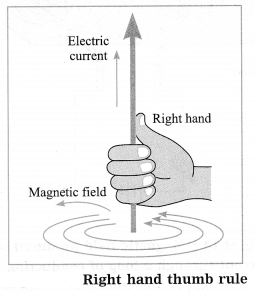Question 29.
With a neat labelled diagram, describe the pattern of magnetic lines of force due to a current through a circular loop. Also explain how the magnetic field depends on the number of turns (n) in the loop.
The pattern of magnetic lines of force due to a current through a circular loop is shown in Figure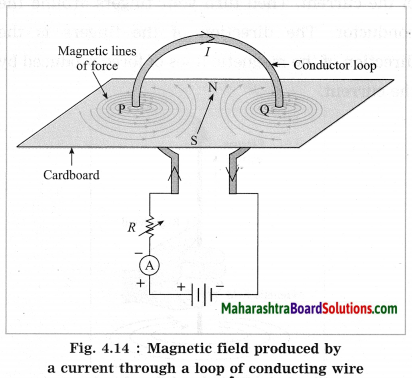(I: Current, R: Resistance, A: Ammeter)
1. It is seen that every point of the loop forms a centre of a large number of concentric magnetic lines of force forming a series. The circles are small near the wire and become large as we move away from the wire. At the centre of the loop, the arcs of these circles appear as straight lines because of very large radius of the circle.

2. The magnetic field produced by a current-carrying wire at a given point is directly proportional to the current through the wire. If the loop has n turns, the field produced is n times that produced by a single turn (assuming that all the turns have practically the same radius and are in the same plane). The reason is the current in each turn has the same direction and the field due to each turn contributes equally to the total field.

Question 30.
Write Fleming’s left hand rule.
Fleming’s left hand rule: The left hand thumb, index finger, and the middle finger are stretched so as to be perpendicular to each other. If the index finger is in the direction of the magnetic field, and the middle finger points in the direction of the current, then the direction of the thumb in the direction of the force on the conductor.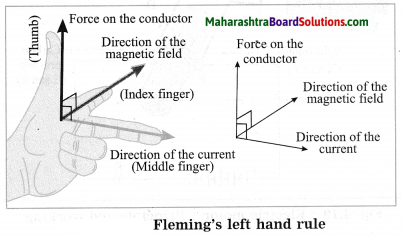[Note: A magnetic field exerts a force on a current-carrying conductor. Electric current is the time rate of flow of electric charge. Thus, a magnetic field exerts a force on a moving charge. This property is used to accelerate charged particles such as protons, deuterons and alpha particles, as well as electrons, to very high energies. A machine used for this purpose is called a charged particle accelerator. It may be linear or circular in design and very big in size. Such high energy particles are used to study the structure of matter. ]

Question 31.
What is electric motor?
A device that converts electric energy into mechanical energy is called an electric motor.

Question 32.
State the principle on which the working of an electric motor is based.
An electric motor works on the principle that a current-carrying conductor placed in a magnetic field experiences a force. In this case, the forces acting on different parts of the coil of the motor produce the rotational motion of the coil.Question 33.
State the uses/applications of an electric motor.
Uses/applications of an electric motor:

1. In domestic appliances such as a mixer, a blender, a refrigerator and washing machine.
2. In an electric fan, a hair dryer, a record player, a tape recorder and a blower.
3. In an electric car, a rolling mill, an electric crane, an electric lift, a pump, a computer and an electric train.

Question 34.
(i) Which principle is explained in this figure?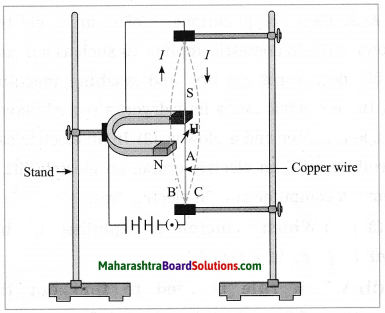(ii) Which rule is used to find out the direction of force in this principle?
(iii) In which machine is this principle used? Draw a diagram showing the working of that machine. (Practice Activity Sheet – 2)
(i) A force is exerted on a current-carrying conductor in the presence of a magnetic field.
(ii) Fleming’s left hand rule is used.
(iii) Electric motor.
Scientifically and technically correct figure.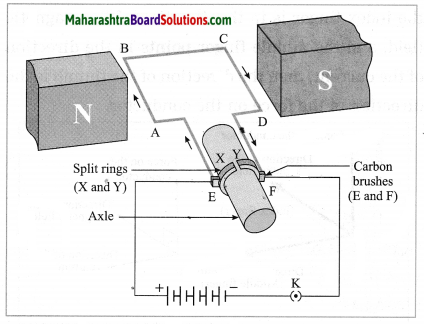Question 35.
Observe the following diagram and answer the questions. (Practice Activity Sheet – 1)
(a) Construction of which equipment does the following diagram show?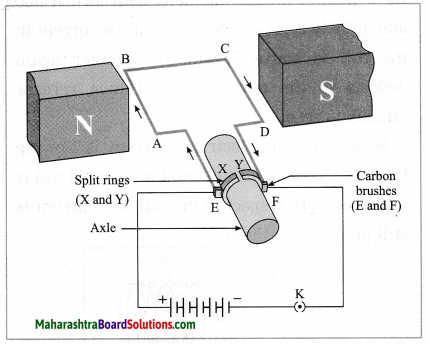(b) On which principle does this equipment work?
(c) According to which law does the coil ABCD rotate?
(d) Write the law in your own words.
(e) Where is this equipment used?
(a) Given diagram shows the construction of an electric motor.

(b) An electric motor works on the principle that a current-carrying conductor placed in a magnetic field experiences a force. In this case, the forces acting on different parts of the coil of the motor produce the rotational motion of the coil.

(c) The rotation of the coil is based on Fleming’s left hand rule.

(d) Fleming’s left hand rule: The left hand thumb, index finger, and the middle finger are stretched so as to be perpendicular to each other. If the index finger is in the direction of the magnetic field, and the middle finger points in the direction of the current, then the direction of the thumb in the direction of the force on the conductor.

(e) Uses / applications of an electric motor: (1) In domestic appliances such as a mixer, a blender, a refrigerator and washing machine. (2) In an electric fan, a hair dryer, a record player, a tape recorder and a blower. (3) In an electric car, a rolling mill, an electric crane, an electric lift, a pump, a computer and an electric train.Question 36.
Study the following principle and answer the questions. (Practice Activity Sheet – 4)
A force is excreted on a current-carrying conductor placed in a magnetic field. The direction of this force depends on both the direction of the current and the direction of the magnetic field. This force is maximum when the direction of the current is perpendicular to the direction of the magnetic field.
(a) By which law can we determine the direction of the force excreted on the current-carrying conductor?
(b) In which electrical equipment is this principle used?
(c) Draw a diagram representing the construction of this equipment.
(d) Write the working of this equipment in brief.
(a) Fleming’s left hand rule.
(b) Electric motor,
(c)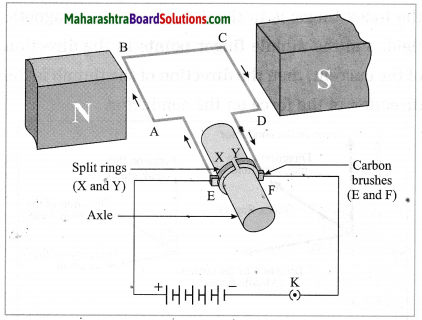(d) Working:
1. When the circuit is completed with a plug key or switch, the current flows in the direction E → A → B → C → D → F. As the magnetic field is directed from the north pole to the south pole, the force on AB is downward and that on CD is upward by Fleming’s left hand rule. Hence, AB moves downward and CD upward. These forces are equal in magnitude and opposite in direction. Therefore, as observed from the side AD, the loop ABCD and the axle start rotating in anticlockwise direction.

2. After half a rotation, X and Y come in contact with brushes F and E respectively and the current flows in the direction EDCBAF. Hence the force on CD is downward and that on AB is upward. Therefore, the loop and the axle continue to rotate in the anticlockwise direction.

3. After every half rotation, the current in the loop is reversed and the loop and the axle continue to rotate in anticlockwise direction.
When the current is switched off, the loop stops rotating after some time.

Question 37.
What is a galvanometer used for? Explain in brief the working of a galvanometer.
Galvanometer is a sensitive device used to detect the presence of current in a circuit as well as to determine the direction of the current in the circuit.

With suitable modification, it can be used to measure charge, current and voltage. Its working is based on the same principle as that of an electric motor. Here, a coil is pivoted (or suspended) between the pole pieces of a magnet and a pointer is connected to the coil. As the coil rotates when a current is passed through it, the pointer also rotates. The rotation of the coil and hence the deflection of the coil is proportional to the current. The pointer deflects on both sides of the Central zero mark depending on’ the direction of the current.

Question 38.
Take a coil AB having 10-15 turns. Connect the two ends of the coil to the galvanometer as shown in Figure. Take a strong bar magnet. (1) Move the north pole of the magnet towards the end B of the coil.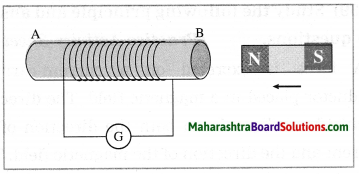Observe the deflection of the pointer in the galvanometer. Note the direction of the deflection (i.e. right or left). (2) Now repeat this with the south pole of the magnet towards the end B of the coil. Again observe the deflection. Note its direction. (3) What will happen if instead of the magnet, the coil is moved? (4) If both the coil and the magnet are kept stationary, do you observe any deflection? (5) Compare the direction of the deflection when the north pole of the magnet is moved towards the end B of the coil with that when the end B of the coil is moved away from the north pole of the magnet. (6) What conclusions do you draw from the observations?
Observations:
The two deflections, in parts (1) and (2) of the experiment, are in the opposite directions.

(3) If instead of the magnet, the coil is moved towards the stationary magnet, the deflection of the pointer in the galvanometer is observed in one direction, while if the coil is moved away from the magnet, the deflection is observed in the opposite direction. The effect of moving the north pole of the magnet towards the coil and the effect of moving the coil towards the north pole of the magnet are the same.

(4) If both the coil and the magnet are kept stationary, no deflection is observed.

(5) The two deflections are in opposite directions.

(6) Whenever there is relative motion of the coil and the magnet, electric potential difference is induced in the circuit which gives rise to, i.e., induces, an electric current in the circuit causing the deflection of the pointer in the galvanometer. The direction of the current and hence that of the deflection of the pointer in the galvanometer depends on which pole of the magnet faces the coil as well as the direction of relative motion.

[Note: If the velocity of the magnet is increased, the induced current increases, and hence the deflection of the pointer in the galvanometer increases.]Question 39.
Take two coils of about 50 turns. Insert them over a nonconducting cylindrical roll as shown in Figure.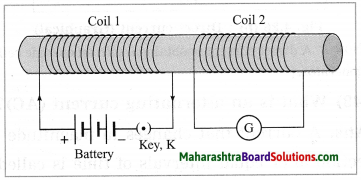(A thick paper roll can be used.) Connect coil 1 to a battery with a plug key K. Connect coil 2 to a galvanometer G. (1) Plug the key and observe the deflection in the galvanometer. (2) Unplug the key and again observe the deflection.
Note your observations. What conclusions do you draw from these observations?
Observations :
1. When the key is plugged, the galvanometer shows a momentary deflection. When the current in coil 1 becomes steady, the galvanometer shows zero deflection, i.e., its pointer returns to the zero mark at the centre of the scale.

2. When the key is unplugged, the galvanometer shows a momentary deflection in the opposite direction relative to that in part (1) of the experiment. When the current in coil 1 becomes zero as the circuit is broken on unplugging the key, the galvanometer shows zero deflection, i.e., its pointer returns to the zero mark at the centre of the scale.

Conclusions:
As the current in coil 1 changes, the magnetic field associated with the current changes. This induces an electric potential difference in coil 2 which gives rise to an electric current and hence the deflection of the galvanometer. The direction of the induced current and hence that of the deflection of the pointer in the galvanometer depends on whether the current through coil 1 increases or decreases with time.

When there is a steady current in coil 1, there is no change in the associated magnetic field and hence no production of induced potential difference in coil 2. In that case there is no current in coil 2 and hence the galvanometer shows zero deflection.

[Note : Coil 1 is called the primary coil while coil 2 is called the secondary coil. This is because when the current through coil 1 is changed, induced current appears in coil 2.]

Question 40.
What is electromagnetic induction? Who discovered it?
The process by which a changing magnetic field in a conductor induces a current in another conductor is called electromagnetic induction. A current can be induced in a conductor either by moving it in a magnetic field or by changing the magnetic field around the conductor. Electromagnetic induction was discovered by Michael Faraday in 1831 and independently by Joseph Henry in 1830.

[Note Michael Faraday (1792-1867), British chemist and physicist, discovered the laws of electrolysis, electromagnetic induction, and a magneto-optical effect now known as the Faraday effect. His discoveries also include benzene and the liquefaction of chlorine. Joseph Henry (1797-1878), US physicist, in addition to the dicovery of electromagnetic induction, invented and constructed the first practical electric motor.]

Question 41.
Whenever the number of magnetic lines of force passing through a coil changes, a current is induced in the coil.

Question 42.
State Fleming’s right hand rule.
Stretch the thumb, the index finger and the middle finger of the right hand in such a way that they are perpendicular to each other. In this position, the thumb indicates the direction of the motion of the conductor, the index finger the direction of the magnetic field, and the middle finger shows the direction of the induced current.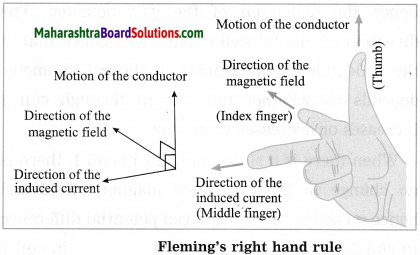[Note The induced current is maximum when the direction of motion of the conductor is at right angles to the magnetic field. ]

Question 43.
Observe the following figure. If the current in the coil A is changed, will some current be induced in the coil B? Explain.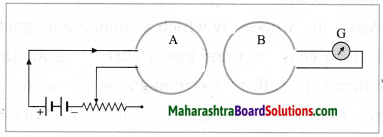If the current in the coil A is changed, there will be some current induced in the coil B.

Explanation:
When the current in the coil A is changed, the magnetic field associated with the current changes. This induces potential difference in the coil B. This gives rise to (i.e., induces) a current in the coil B. The greater the rate at which the current in the coil A is changed with respect to time, the greater is the current induced in the coil B as can be seen from the deflection of the pointer in the galvanometer. This phenomenon is known as electromagnetic induction.Question 44.
What is a direct current (DC)?
A nonoscillatory current that flows only in one direction is called a direct current (DC). It can change in magnitude, but its direction remains the same. [Fig. 4.26 (a) and (b)]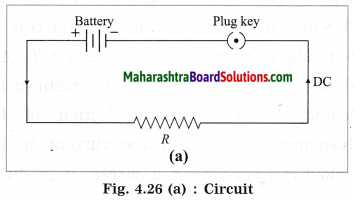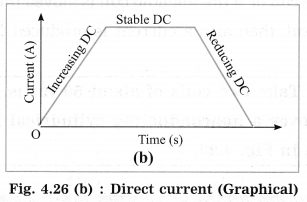[Note: A direct current is obtained with an electric cell or a DC generator.]

Question 45.
What is an alternating current (AC)?
A current that changes in magnitude and direction after equal intervals of time is called an alternating current (AC) (Fig. 4.27).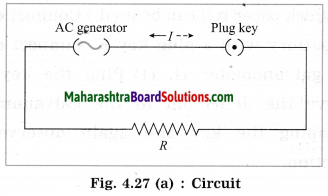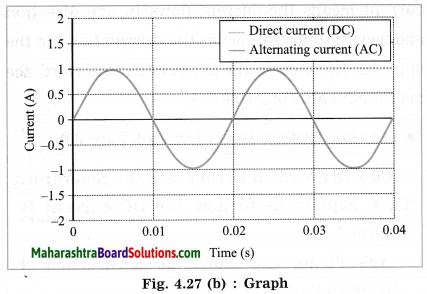Electric current changes sinusoidally with time. Electric current and potential difference are shown by the symbol ~.
[Note: An alternating current is obtained with an AC generator.]

Question 45.
What is the value of frequency of AC in India?
In India, the value of frequency of AC is 50 hertz.

Question 46.
What is the periodic time of AC in India?
In India, the periodic time of AC is 0.02 s (=$$\frac{1}{50}$$s)

Question 47.
State one advantage of AC over DC.
One advantage of AC over DC is that electric power can be transmitted over long distances without much loss of energy.Question 48.
Name two appliances/devices in which a direct current is used.
A direct current is used in a portable electric torch and radio.
[Note A Direct current is also in an electric bell, a wall clock, to prepare an electromagnet, for electrolysis, etc. ]

Question 49.
Name two appliances/devices in which an alternating current is used.
(OR)
State any two uses of an AC generator.
An alternating current is used in an electric heater and a refrigerator.
[Note Alternating current is also used in an electric iron, a washing machine, an electric mixer, a food processor, an air-conditioner, an electric fan, etc.]

Question 50.
What is (1) an electric generator (2) an AC generator (3) a DC generator?
(1) A device which converts mechanical energy into electric energy is called an electric generator.
(2) A generator which converts mechanical energy into electric energy in the form of an alternating current (AC) is called an AC generator.
(3) A generator which converts mechanical energy into electric energy in the form of a direct current (DC) is called a DC generator.

Question 51.
State the principle on which the working of an electric generator is based.
The working of an electric generator is based on the principle of electromagnetic induction. When the coil of an electric generator rotates in a magnetic field, a current is induced in the coil. This induced current then flows in the circuit connected to the coil.
[Note An external agency is needed to rotate the coil of an electric generator.]

Question 52.
Show graphically variation of AC with time. Explain the nature of the graph.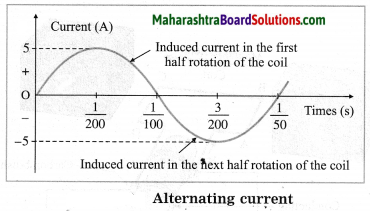In this case, the frequency of the alternating current (AC) produced is 50 Hz. The coil completes 50 rotations every second. The time for one rotation of the coil is $$\frac{1}{50}$$ second. It is called the periodic time or simply the period of AC. Positive current means the current flows in one direction and negative current means the current flows in the opposite direction in the external circuit. Here, the maximum value of AC is 5 A.

Question 53.
Observe the figure and answer the following questions.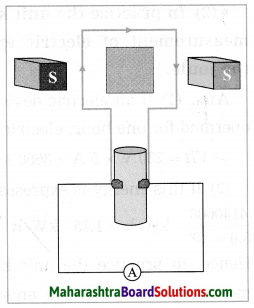(a) Identify the machine shown in the figure.
(b) Write a use of this machine.
(c) How transformation of energy takes place in this machine. (Practice Activity Sheet – 3)
(a) The instrument shown in the figure is generator.
(b) This machine is used to generate electricity.
(c) The generator generates electricity through following transformation:
Mechanical Energy → Electrical EnergyQuestion 54.
Observe the following figure. Which bulb will fuse? (Practice Activity Sheet – 4)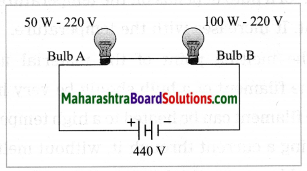Bulb A.

Give scientific reasons:

Question 1.
In an electric iron, the coil of high resistance is kept between mica sheets.
(1) Mica is a bad conductor of electricity and good conductor of heat.
(2) In an electric iron, the coil of high resistance is kept between mica sheets so that there is no electrical contact between the coil and the heavy metal block of the iron though there is heat transfer. This protects the user from getting an electric shock.

Question 2.
The material used for fuse has low melting point.
OR
A fuse should be made of a material of low melting point.
1. A fuse is used to protect a circuit and the appliances connected in the circuit by stopping the flow of an excessive electric current. For this, a fuse is connected in series in the circuit.

2. When the current in the circuit passes through the fuse, its temperature increases. When the current exceeds the specified value, the fuse must melt to break the circuit. For this, the material used for a fuse has low melting point.

Distinguish between the following:

Question 1.
Direct current and Alternating current.
Direct current:

1. Direct current flows only in one direction.
2. It cannot be used for large scale of electricity for household purpose.

Alternating current:

1. Alternating current reverses its direction periodically with time.
2. It is used in household electrical appliances such as an electric heater, an electric iron, a refrigerator, etc.Question 2.
Electric motor and Electric generator.
Electric motor:

1. A battery is used in an electric motor to pass a current through the coil.
2. In this case, a current-carrying coil is set in rotation due to the magnetic field.
3. Split rings are used in an electric motor.
4. In this case, electric energy is converted into mechanical energy.

Electric generator:

1. A battery is not used in an electric generator.
2. In this case, a potential difference and hence a current is produced when the coil is set into rotation in the magnetic field by an external agent.
3. Rings used in an AC generator are not split.
4. In this case, mechanical energy is converted into electric energy.

Solve the following examples/numerical problems:

Question 1.
An electric bulb is connected to a source of 250 volts. The current passing through it is 0.27 A. What is the power of the bulb?
Solution:
Data: V = 250 V, I = 0.27 A, P = ?
P = VI
= 250 V × 0.27 A
= 67.5 W
The power of the bulb = 67.5 W.

Question 2.
If a bulb of 60 W is connected across a source of 220 V, find the current drawn by it.
Solution:
Data: P = 60 W, V = 220 V, I = ?
P = VI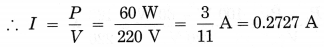The current drawn by the bulb
= $$\frac{3}{11}$$ A = 0.2727 A

Question 3.
A bulb of 40 W is connected across a source of 220 V. Find the resistance of the bulb.
Solution:
Data: P = 40 W, V = 220 V, R = ?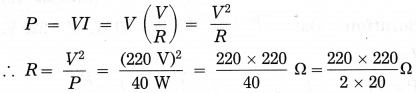= 40 × 110 Ω = 1210 Ω
The resistance of the bulb = 1210 Ω

Question 4.
If the current passing through a bulb is 0.2 A and the power of the bulb is 20 W, find the voltage applied across the bulb.
Solution:
Data: I = 0.2 A, P = 20 W, V = ?
P = VI
∴ V = $$\frac{P}{I}=\frac{20 \mathrm{W}}{0.2 \mathrm{A}}$$
= 100 V
The voltage across the bulb = 100 V.Question 5.
Two tungsten bulbs of power 50 W and 60 W work on 220 V potential difference. If they are connected in parallel, how much current will flow in the main conductor? (March 2019)
Solution:
Data: P1 = 50 W, P2 = 60 W, V = 220 V, I = ?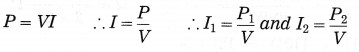Current in the main conductor,
I = I1 + I2 ……….(parallel combination)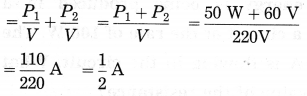= 0.5 A

Question 6.
An electric iron rated 750 W is operated for 2 hours/day. How much energy is consumed by the electric iron for 30 days?
Solution:
Data: P = 750 W, t = 2$$\frac{\text { hours }}{\text { day }}$$ for 30 days
The energy consumed = Pt = 750 × 2 × 30
= 1500 × 30
= 45000 W·h
= 45 kW·h
The energy consumed by the electric iron for 30 days = 45 kW·h.

Question 7.
If a TV of rating 100 W operates for 6 hours per day, find the number of units consumed in a leap year.
Solution:
Data: P = 100 W, t = 6$$\frac{\text { hours }}{\text { day }}$$ × 366 days
= 2196 hours
1 unit = 1 kW·h = 1000 W·h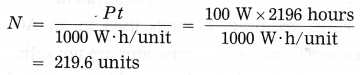219.6 units are consumed in a leap year.

Question 8.
An electric appliance of rating 300 W is used 5 hours per day in the month of March. Find the number of units consumed.
Solution:
Data: P = 300 W, t = 5$$\frac{\text { hour }}{\text { day }}$$ × 31 days
= 155 hours, 1 unit = 1 kW·h = 1000 W.h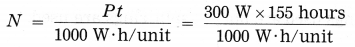= 46.5 units
46.5 units are consumed in the month of March.

Question 9.
A washing machine rated 300 W operates one hour/day. If the cost of a unit is ₹ 3.00, find the cost of the energy to operate the washing machine for the month of March.
Solution:
Data: P = 300 W, ₹ 3.00 per unit,
t = 1 $$\frac{\text { hour }}{\text { day }}$$ × 31 days = 31 hours, 1 unit = 1 kW·h
= 1000 W·h, cost of the energy = ?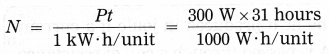Cost = 9.3 units × ₹ 3.00 per unit = ₹ 27.9.
The cost of the energy to operate the washing machine for the month of March = ₹ 27.9.Question 10.
Find the heat produced in joule if a current of 0.1 A is passed through a coil of resistance 50 Ω for two minutes. Keeping other conditions the same if the length of the wire is reduced to the original length (by cutting 4 the wire), what will be the heat produced?
Solution:
Data: I = 0.1 A, R = 50 Ω, t = 2 minutes = 2 × 60 s = 120 s, H = ?
H = I2Rt = (0.1A)2 × 50 Ω × 120 s
= 0.01 × 50 × 120 J = 60 J
Heat produced = 60 joules.
In the second case, the resistance of the wire will be $$\frac{50 \Omega}{4}$$
Hence, the heat produced = $$\frac{60 \mathrm{J}}{4}$$ = 15 J.

Question 11.
Calculate the heat produced in calorie when a current of 0.1 A is passed through a wire of resistance 41.8 Ω for 10 minutes.
Solution:
Data: I = 0.1 A, R = 41.8 Ω, t = 10minutes
= 10 × 60 s = 600 s, H = ?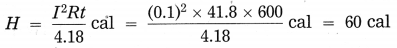Heat produced = 60 calories.

Question 12.
A potential difference of 250 volts is applied across a resistance of 1000 Ω in an electric iron. Find (1) the current (2) the heat produced in joule in 12 seconds.
Keeping other conditions the same, if the length of the wire in the iron is reduced to half the original length (by cutting the wire), what will be the current and heat produced?
Solution:
Data: V = 250 V, R = 1000 Ω, t = 12 s,
I = ? H = ?
(1) V = IR
∴ I = $$\frac{V}{R}=\frac{250 \mathrm{V}}{1000 \Omega}$$ = 0.25 A
The current through the resistance = 0.25 A.
(2) H = I2RT
= (0.25 A)2 × 1000 Ω × 12 s
= ($$\frac{1}{4}$$ × 1000) × ($$\frac{1}{4}$$ × 12) J
= 250 × 3J = 750 J
H = VIt = 250 V × 0.25 A × 12s = 250 × 3J = 750 J
The heat energy produced in the resistance in 12 seconds = 750 joules.

On cutting the wire, the resistance of the wire will become half the initial resistance. Hence, the current will become double the initial current as I = V/R and V is the same in both the cases. Therefore, the current in the wire will be 0.25 A × 2 = 0.5 A. (Hence, the heat produced will be VIt = 250 V × 0.5 A × 12 s = 250 × 6 J = 1500 J.)

Question 13.
A potential difference of 100 V is applied across a resistor of resistance 50 Ω for 6 minutes and 58 seconds. Find the heat produced in (i) joule (ii) calorie.
Solution:
Data: V = 100 V, R = 50 Ω, t = 6 minutes and 58 seconds = (6 × 60 + 58) s = (360 + 58)
= 418 s, H = ?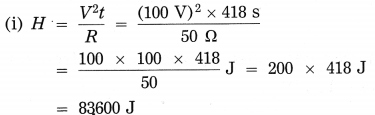Heat generated = 83600 joules.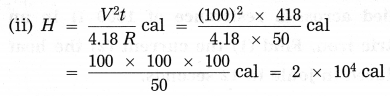Heat produced = 2 × 104 calories.

Numerical Problems For Practice:

Question 1.
When the voltage applied across a bulb is 200 V, the current passing through the bulb is 0.1 A. Find the power of the bulb.
20 W

Question 2.
A bulb of 100 W is connected across a source of 200 V. Find the current drawn by it.
0.5 AQuestion 3.
A bulb of 60 W is connected across a source of 240 V. Find the resistance of the bulb.
960 Ω

Question 4.
If the current passing through a bulb is 0.15 A and the power of the bulb is 30 W, find the voltage applied across the bulb.
200 V

Question 5.
An electric appliance of rating 800 W is used 4 hours per day in the month of December. Find the number of units consumed.
99.2 units

Question 6.
An electric appliance rated 400 W is used 5 hours per day in the month of June. If the cost of a unit is ₹ 3.00, find the energy bill for June.
₹ 180

Question 7.
An electric bulb rated 60 W is used 10 hours per day for 20 days. If the cost of a unit is ₹ 3.00, find the energy bill.
₹ 36

Question 8.
Two electric bulbs rated 60 W and 40 W respectively are used 5 hours per day for 20 days. If the cost of a unit is ₹ 4.00, find the cost of the energy used.
₹ 40

Question 9.
Find the heat produced in joule if a current of 0.1 A is passed through a coil of resistance 25 Ω for one minute.
15 J

Question 10.
Calculate the heat produced in calorie when a current of 0.1 A is passed through a wire of resistance 41.8 Ω for 5 minutes.
30 calories

Question 11.
Calculate the heat produced in calorie when a current of 0.2 A is passed through a wire of resistance 41.8 Ω for 10 minutes.
240 calories

Question 12.
Find the heat produced in calorie when a current of 0.2 A is passed through a wire of resistance 20.9 Ω for 10 minutes.
120 calories

Question 13.
A potential difference of 100 V is applied across a wire of resistance 50 Ω for one minute. Find the heat produced in joule.
1.2 × 104 joules

Question 14.
A potential difference of 100 V is applied across a wire for two minutes. If the current through the wire is 0.1 A, find the heat produced in joule.